# Annex X.2 - EXAMPLES OF SYSTEMIC DISTRIBUTIONS

## X.2.1 Distributions with integers support

Some of the most used distributions are the ones whose support is made-up from ordered and continuous intervals of the integers set {Z}, or natural numbers set {N}. For example, we shall consider a function-type distribution, such as: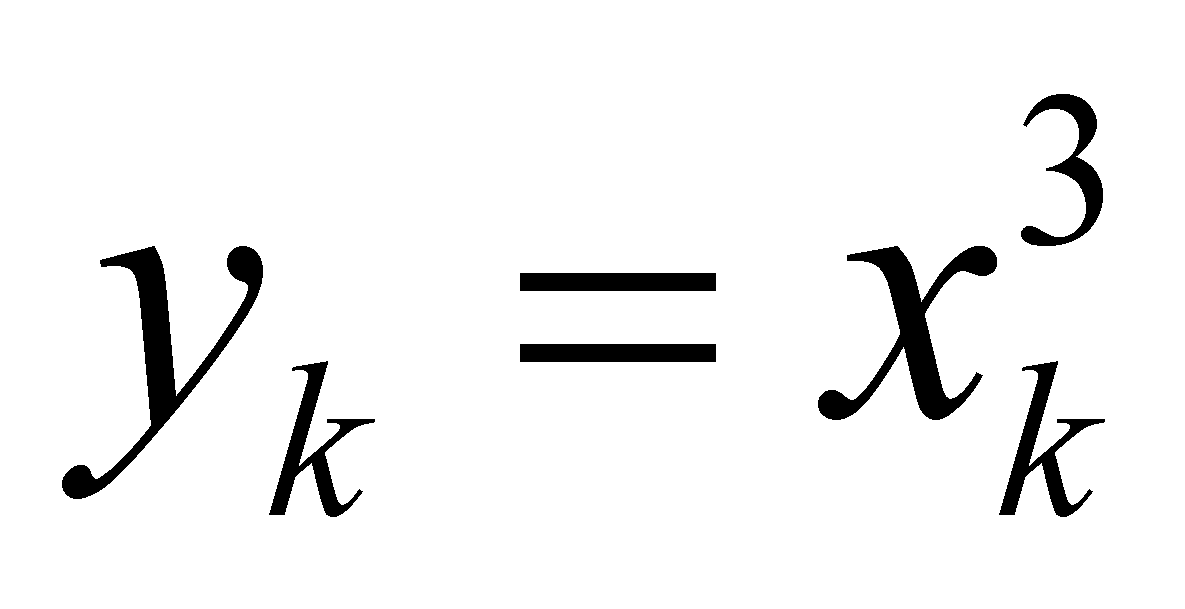(X.2.1.1)

for having non-null and non-even second-rank derived distributions. We are now reminding to the reader that according to the specific notation used in this paper for the calculus with finite differences (where the notation with the reversed rank sign is being used for preventing it to be mistakenly considered as an exponent), the posterior finite difference (which may be also named on the right) between the posterior element yk+1 and the local reference element from series yk is: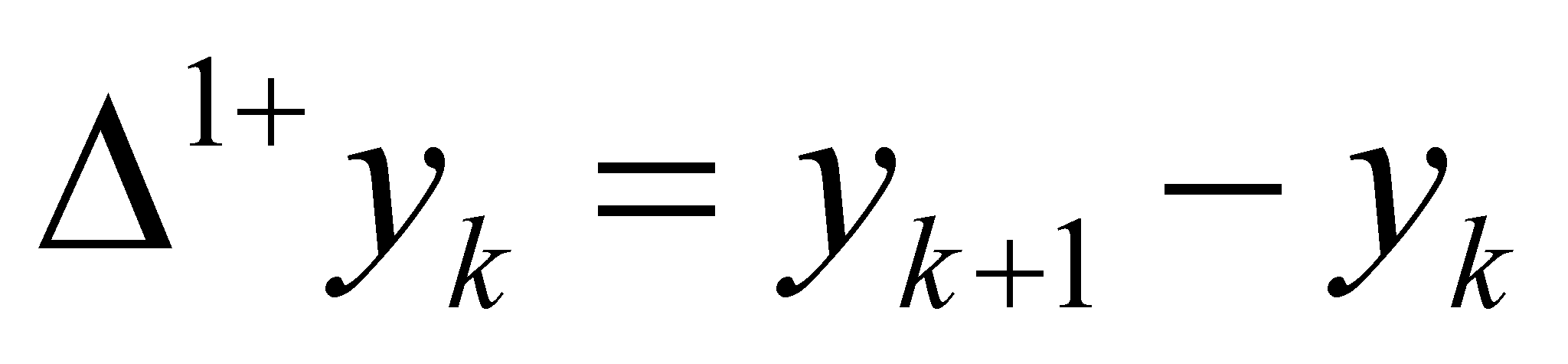(X.2.1.2)

and the anterior finite difference (or on the left against the same reference element yk) is: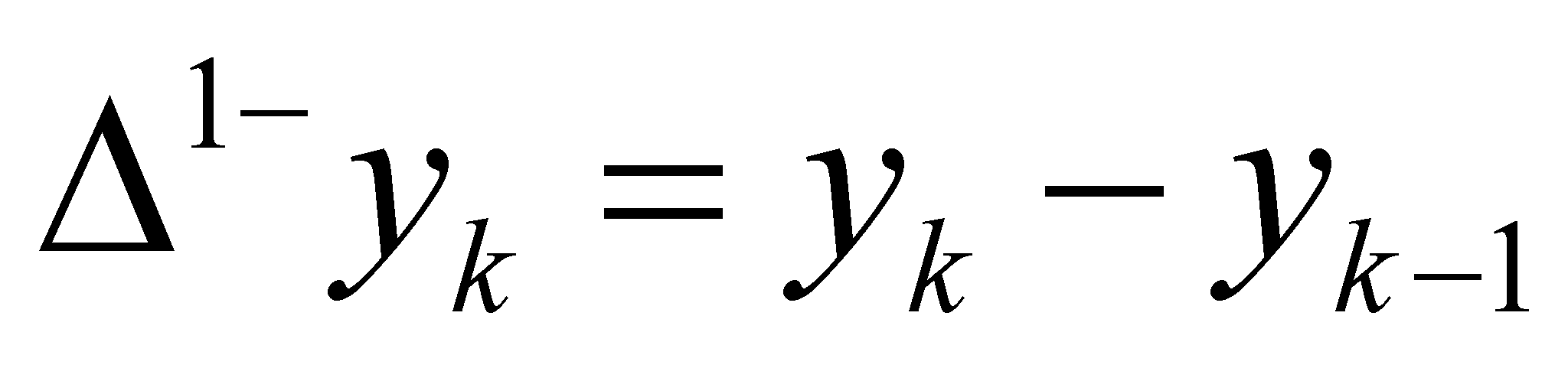(X.2.1.3)

the same for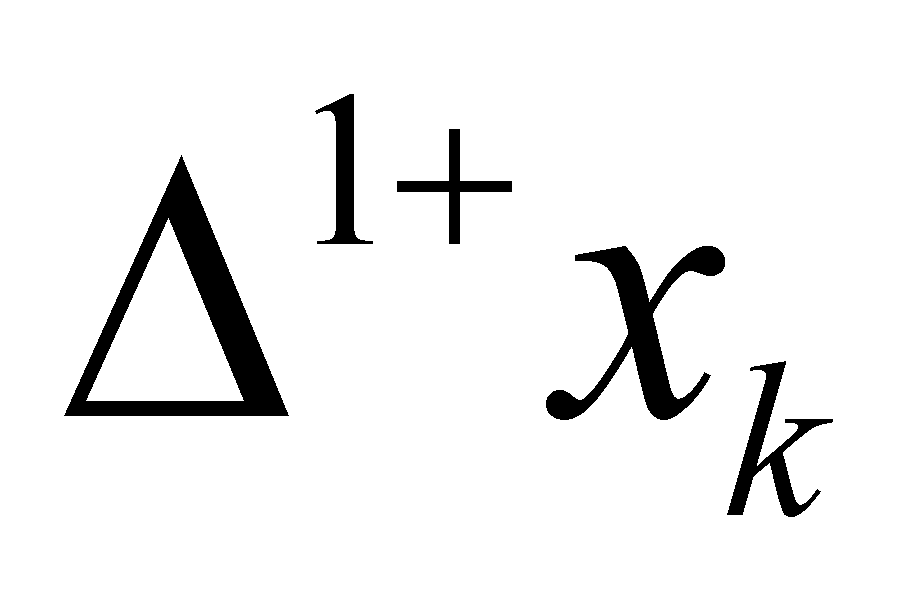or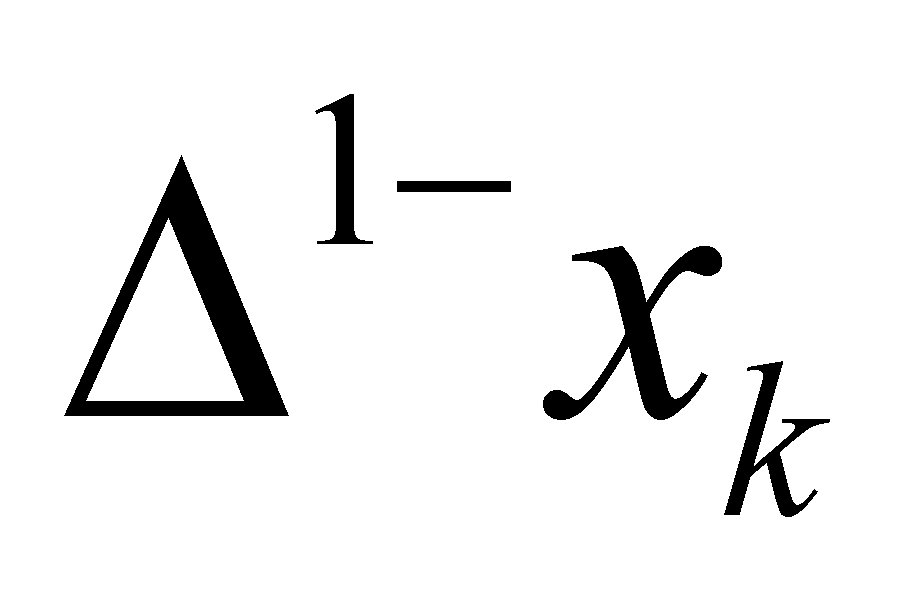, and: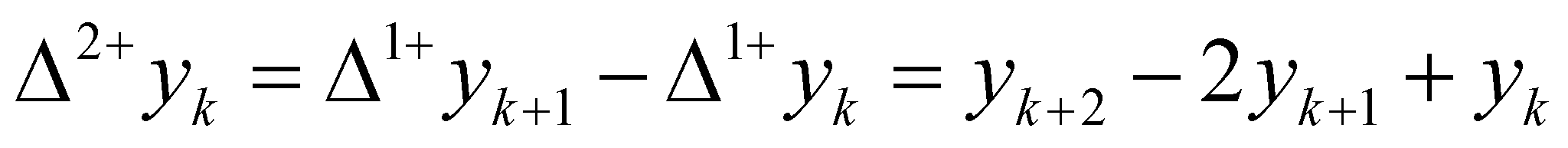(X.2.1.4)

respectively: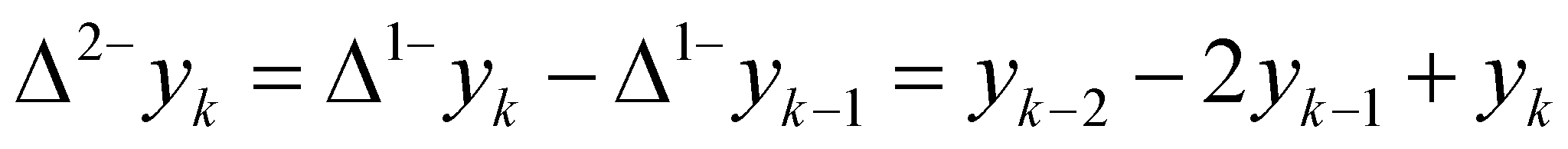(X.2.1.5)

so on.

By looking at the Table 1, one may observe that in the first four columns we are dealing with a primary distribution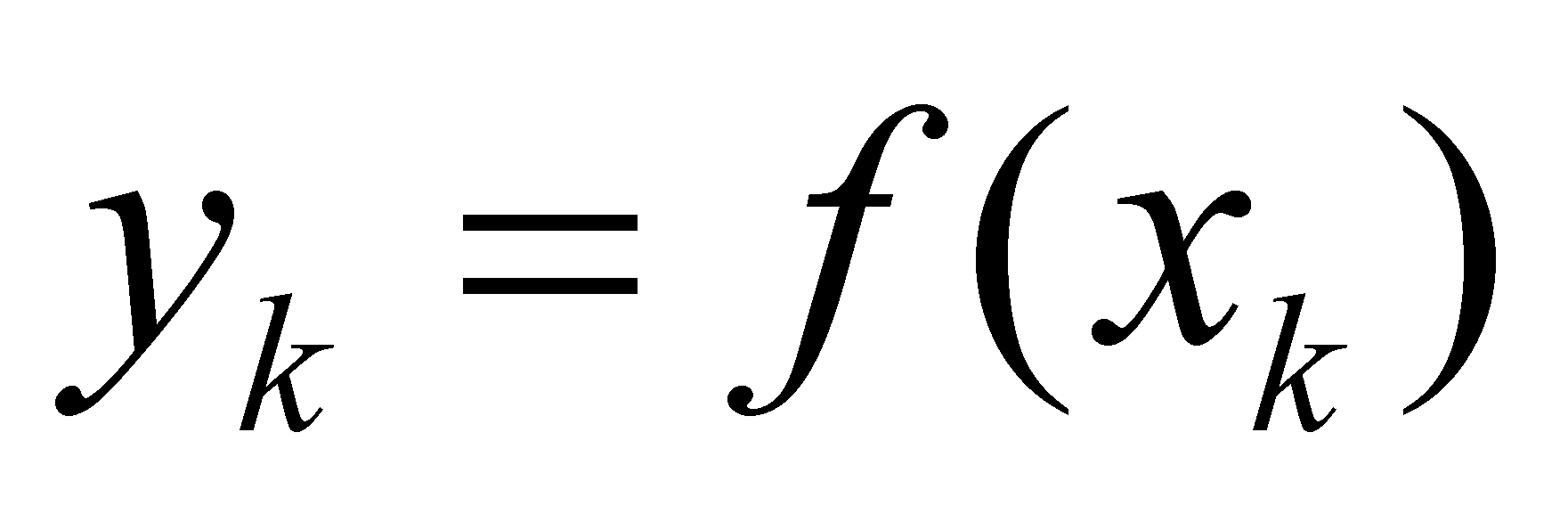, where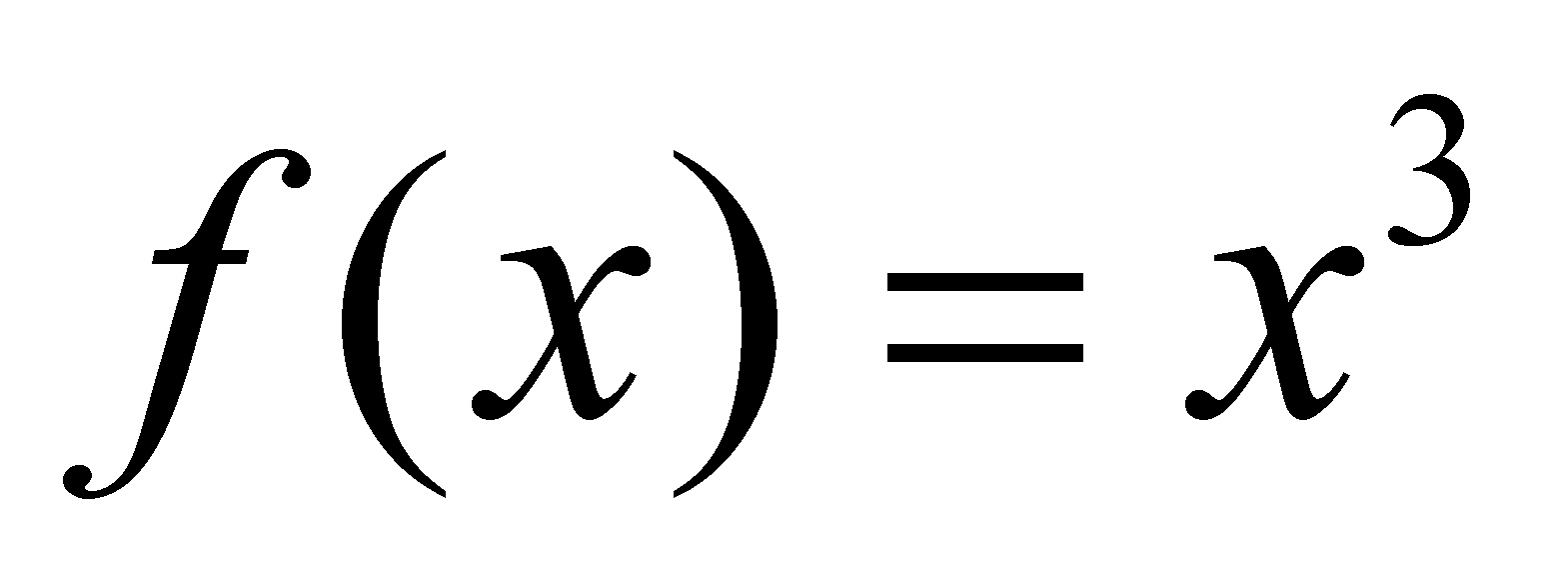, in the columns 5,6,7 and 8, we may find a first rank derived distribution of the primary distribution, and in the following columns, the derived distributions of upper rank (II and III). If in case of this type of discontinuous distributions, a derivative under an algebraic meaning cannot be conceived, we may use instead, with no restrictions, the more general term (introduced by the objectual philosophy) known as density of distributions, which is a term applicable also in the algebraic case (but only by some compulsory conditions, see annex X.3). The table also shows that the two series of support objects (series of the singular values for the primary distribution and series of the elementary variations for the derived distributions) do not have the same number of elements (index values k, m1, m2, m3), but both types of series are using the same value xk as a local reference for the determination of posterior or anterior finite difference (the equivalent of the variations towards the right or left in case of the differential calculus).

It may be also noticed that the elementary support intervals x have all the same size and there is no finite difference (of any rank) between these intervals and consequently, all the elements of the derived distributions, regardless their rank, shall have the same support, that is x. As for the first rank derived distribution, the posterior density (the only one which is computed in Table 1) against the same reference element xk which was above mentioned, shall be: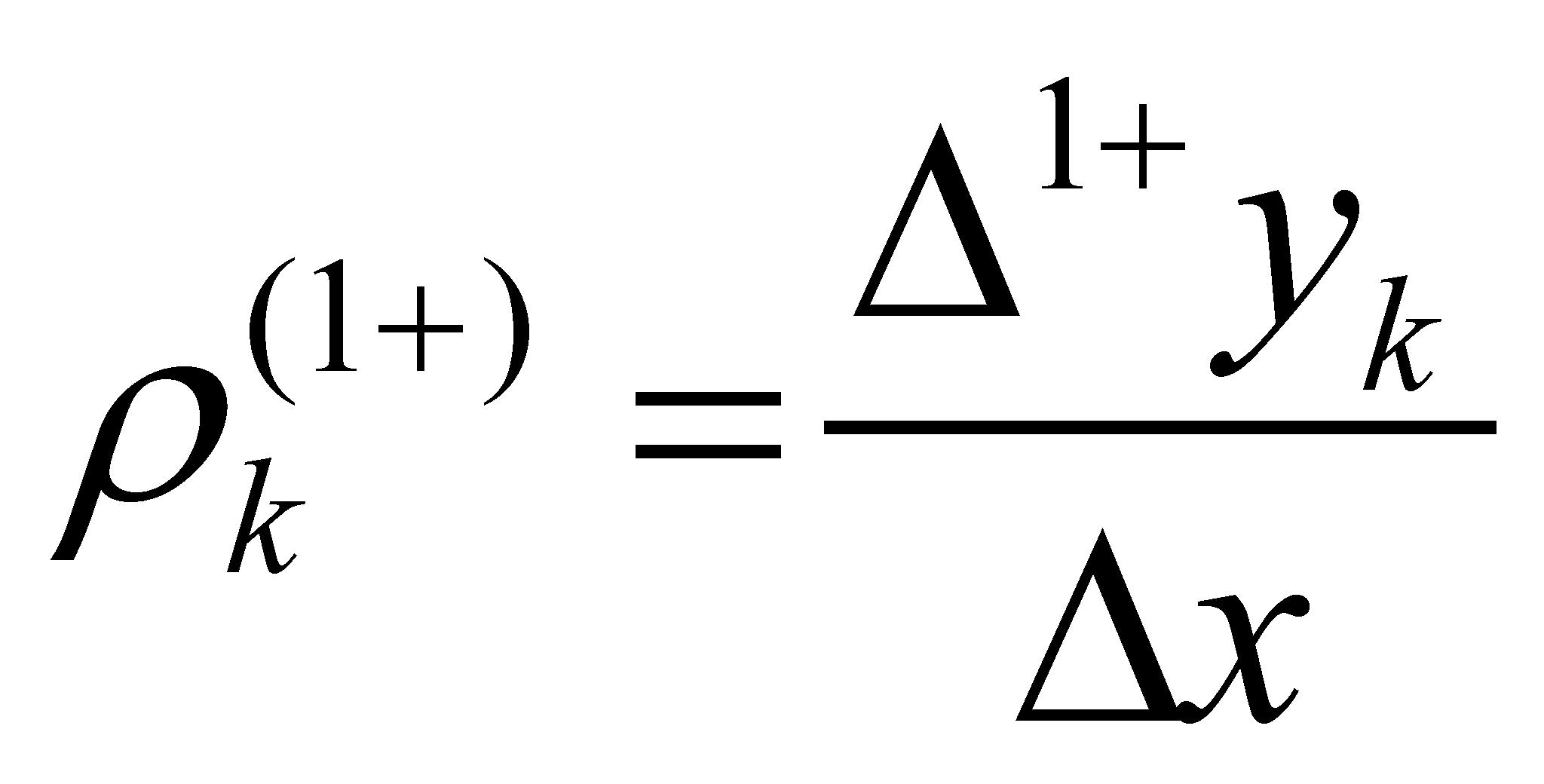(X.2.1.6)

where, because x is the same, regardless the value of k, xk was no longer written.

In the actual case of the primary distribution given by the relation X.2.1.1, and by replacing the values given by X.2.1.2 and X.2.1.6 we shall get: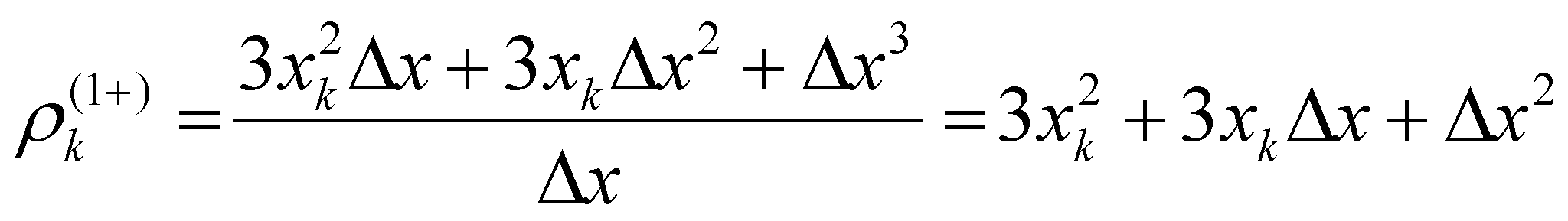(X.2.1.7)

relation which complies perfectly with the values written in the table below.

Table 1

 1 2 3 4 5 6 7 8 9 10 11 12 13 14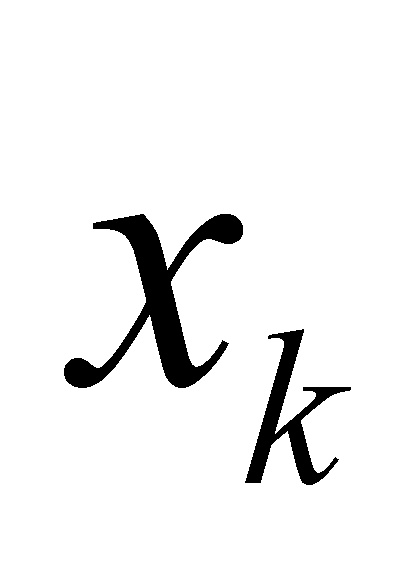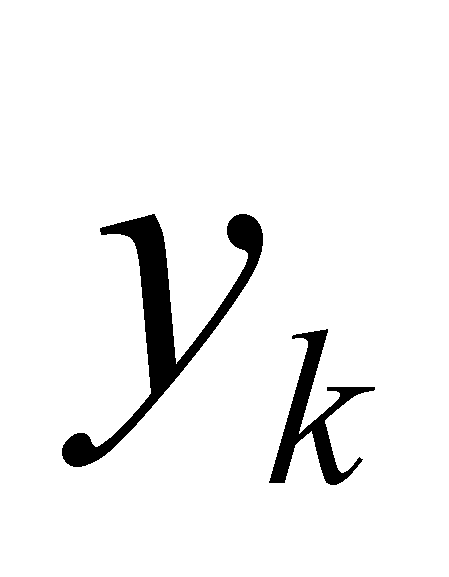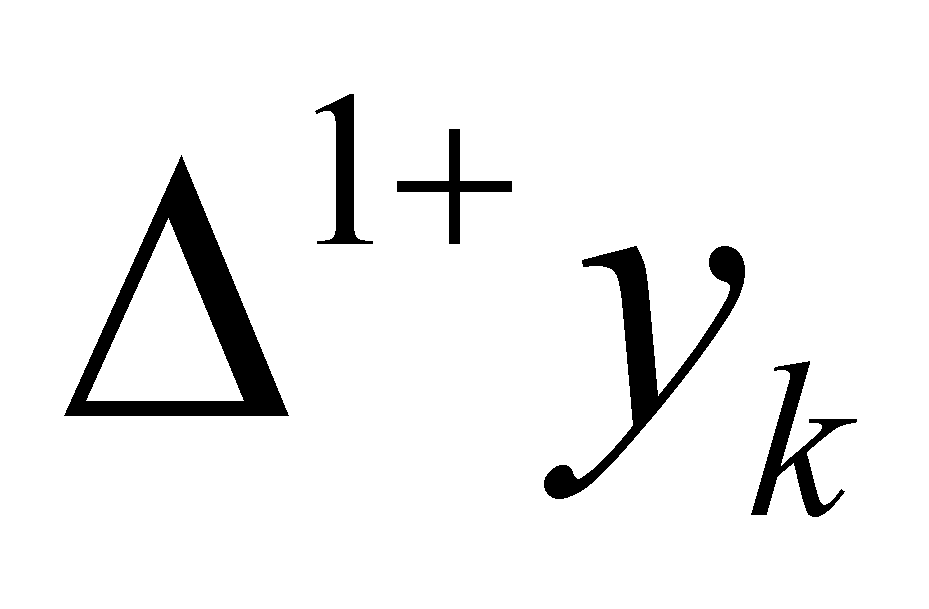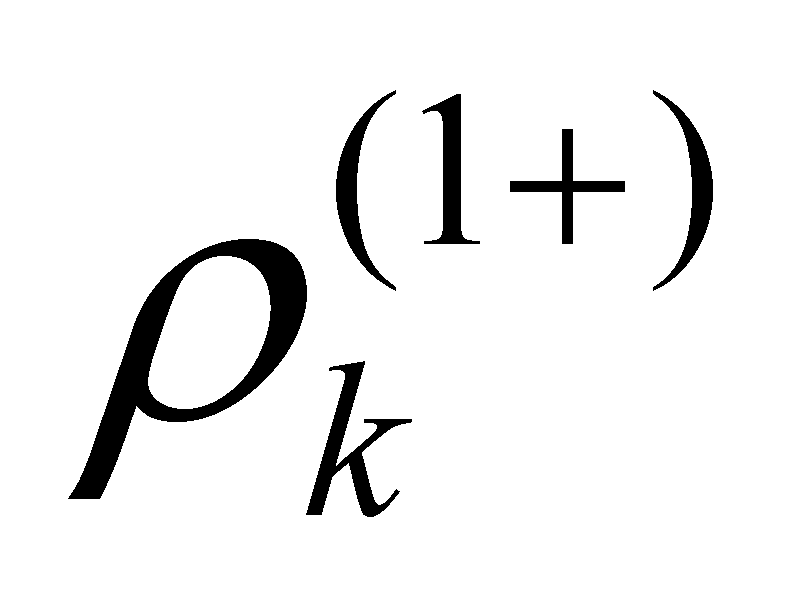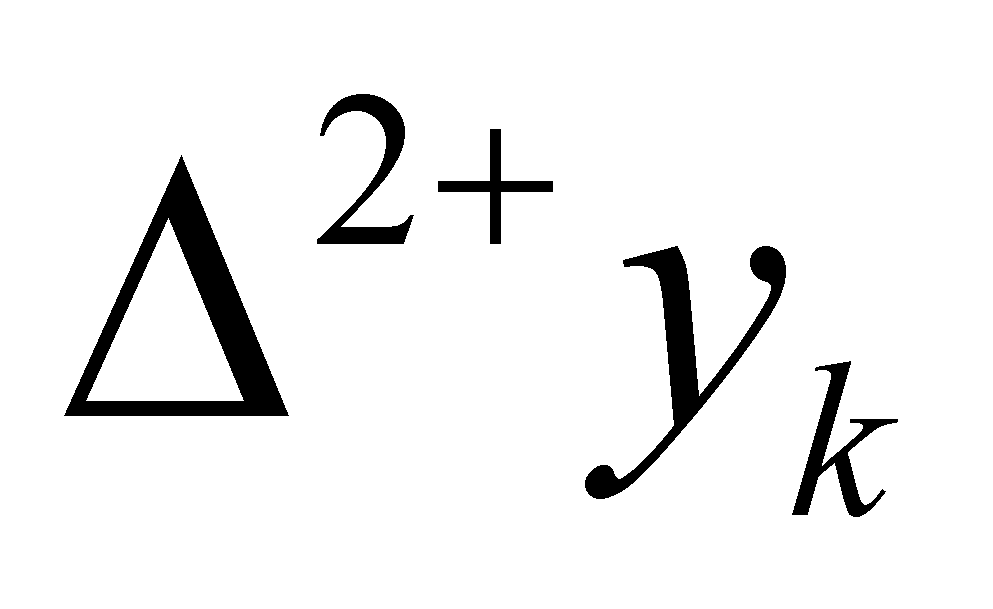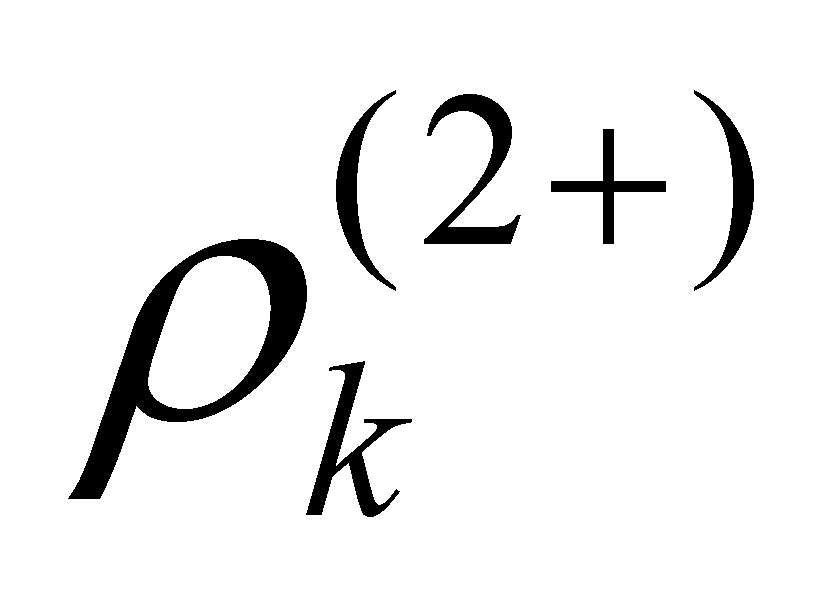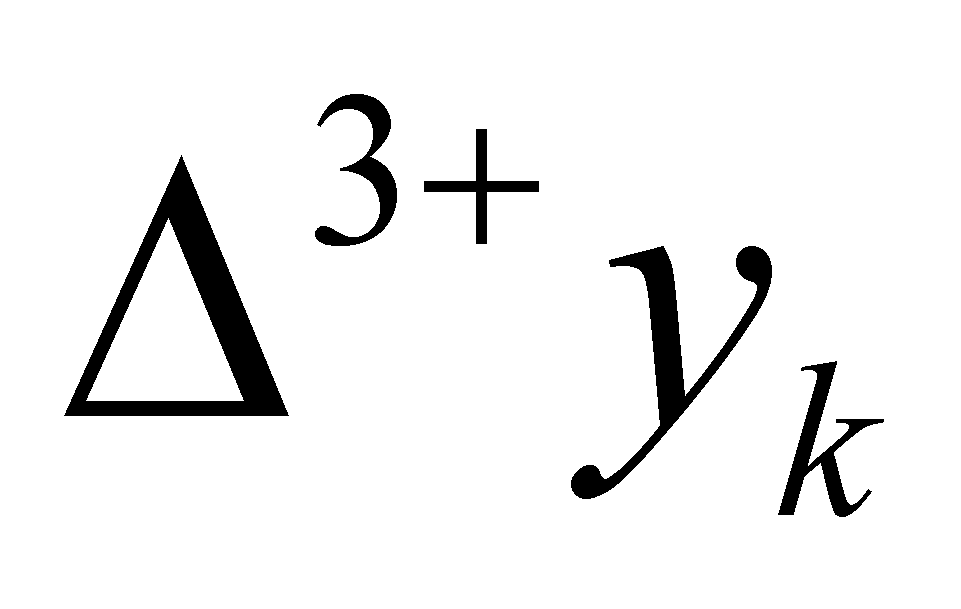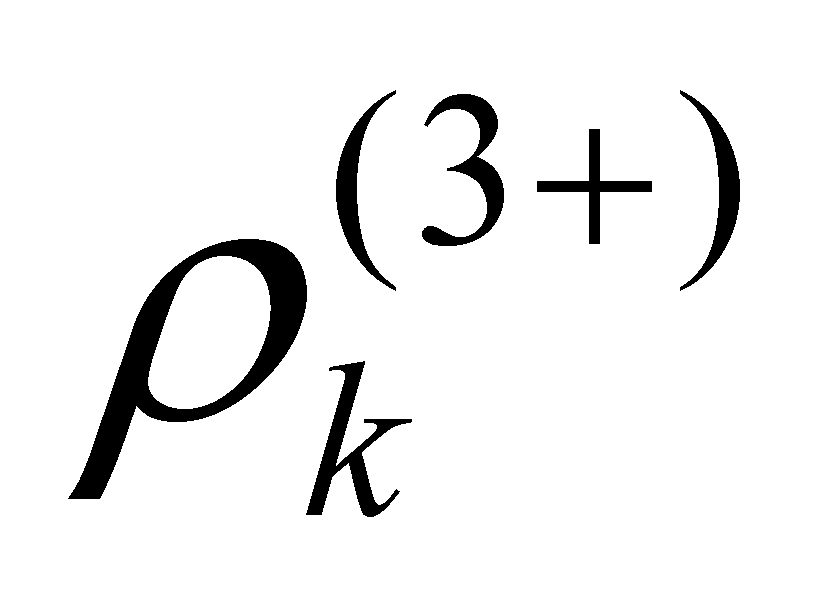1 0 0 1 1 1 1 2 1 1 1 1 6 6 2 1 7 7 1 6 6 3 2 8 4 2 12 12 3 1 19 19 2 6 6 4 3 27 9 3 18 18 4 1 37 37 3 6 6 5 4 64 16 4 24 24 5 1 61 61 4 6 6 6 5 125 25 5 30 30 6 1 91 91 5 6 6 7 6 216 36 6 36 36 7 1 127 127 8 7 343 49

The values yk are (as we have mentioned in chapter 4) objects which belong to the processual class S0, and the valuesare objects which belong to the processual class S1. If the difference between two successive states S0 distributed (assigned) on an elementary support interval Δx is an element of the first rank derived distribution, the difference between two successive states S1 distributed on the same interval Δx shall be the element of the second rank derived distribution, whose density is: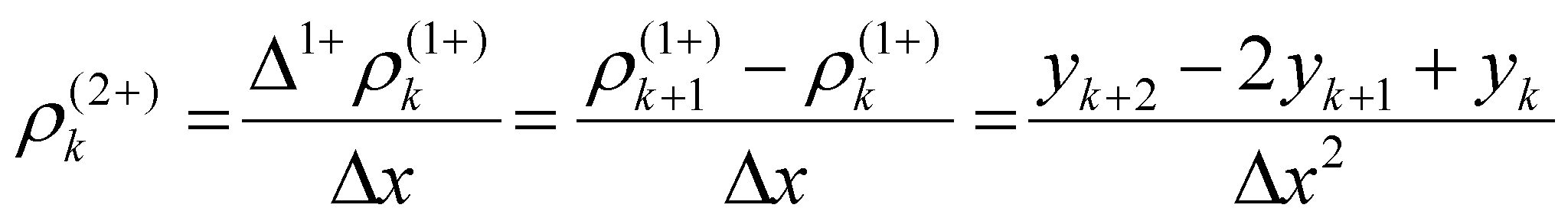(X.2.1.8)

By also making replacements in this case, the actual values given by the relations X.2.1.1, X.2.1.2 and X.2.1.6 within X.2.1.8, we shall finally get: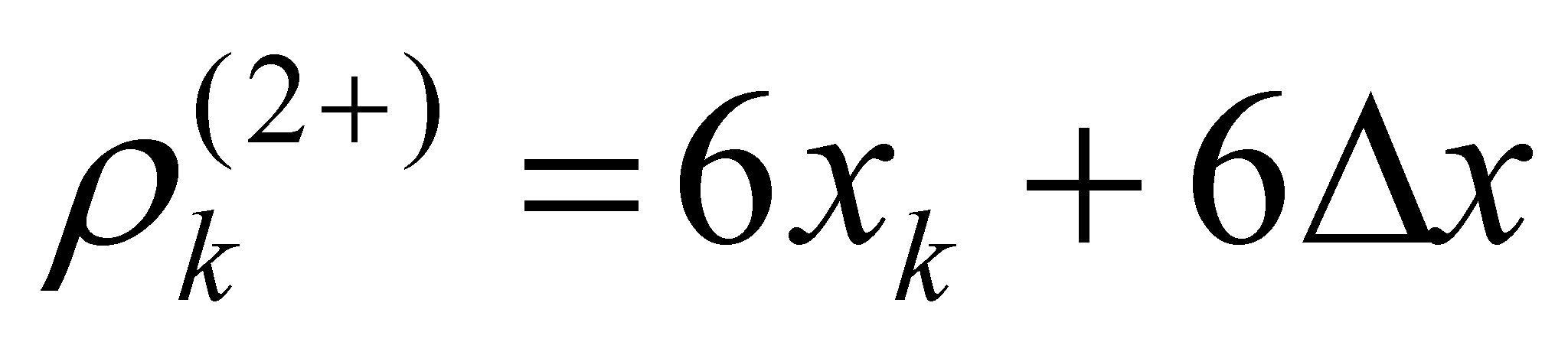(X.2.1.9)

which once again, complies perfectly with the values from Table 1.

This example of a concrete distribution with a support made-up from integers aimed to clearly emphasize the accuracy of the general relations X.2.1.6 and X.2.1.8, as well as the concrete relations X.2.1.7 and X.2.1.9, accurate and valid relations for any kind of amount Δx of the elementary support interval. Those who would neglect in some circumstances the terms which contain Δx (according to the classic differential calculus) have this right, but they will reach to an approximate result, with the error in proportion to the amount Δx. The amountsandare the equivalent of the first and second rank local derivatives (right) from the classic differential calculus.